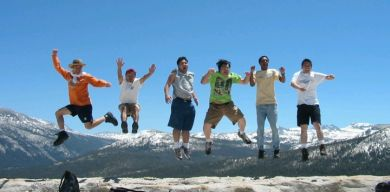Question

# If everyone in China jumped off a chair at the same time, would it cause an earthquake?

Say there are 1.2 billion people in China, and that each one weighs on average 50 kg (140 lbs), and that Chinese chairs are 50 cm (20 inches) high. The total weight of the Chinese nation would then be 60 × 109 kg, or 60 million tons. If an ‘object’ of this sort of weight fell half a metre it would undoubtedly create a fair-sized earthquake. By way of comparison, Esja, the basalt mountain that overlooks Reykjavík, weighs about 2430 tons per square metre, and so it would need about a 24,700 square metre chunk out of Esja (i.e. about 160 m × 160 m) to get up to the combined weight of the Chinese population.

The formula for calculating the potential energy of an object with a given mass m at a given height h under the accelerating force of gravity g is E = mgh. Using this formula we can calculate the energy released by a half metre jump by the entire population of China as follows:
E = 60 × 109 kg × 9.8 ms-2 × 0.5 m = 3 × 1011 joules

If all this energy were converted into earth tremors it would cause an earthquake of force 4.3 on the Richter scale. The relationship between energy (E) and the force of an earthquake (M) is given by the formula:
log E = 5.24 + 144 × M
You would get the greatest effect if all the people in China were brought together in one place, each with their own chair. If we allow them one square metre each, this would require an area of 1200 sq km (470 square miles), corresponding to a square with sides of 35 km (21 miles). The resulting earthquake would certainly register on measuring devices but would not cause any appreciable damage.Some have already started to practice.

Translated by Nicholas Jones.

Picture:: Photo Gallery

## Um þessa spurningu

### Dagsetning

Published 6.3.2005

### Citation

Ritstjórn Vísindavefsins. „If everyone in China jumped off a chair at the same time, would it cause an earthquake? “. The Icelandic Web of Science 6.3.2005. http://why.is/svar.php?id=4809. (Skoðað 2.12.2023).